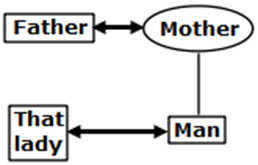## RRB General Intelligence MCQ’s (Set – 1)

Dear Aspirants,
Welcome to Mentor for Bank Exams. Railway exam will be conducted in the month of April/May 2018 as per the official notification.Here we are giving the important General Intelligence MCQ’s for upcomming RRB ALP/Technician and RRB Group D in the exam oriented new pattern, these will help you to score good in Reasoning section.
1. Select the missing number from the given responses.
456     22       434
268     29       239
194     ?          121
a) 32
b) 54
c) 73
d) 86
Explanation: 456 - 434 = 22
268 - 239 = 29
194 - 121 = 73.
2. Pointing towards a lady in a photograph a man said "she is the only daughter-in-law of my Mother's husband". How that lady relates to that man?
a) Aunt
b) Mother
c) Wife
d) Sister
Explanation:3. A word is represented by only one set of number is given in any one of the alternatives. The sets of number given in the alternatives are represented by two classes of alphabets as in two matrices given below. The column and rows of matrix I are numbered from 0 to 4 and that of matrix II are numbered from 5 to 9.A letter from these matrices can be represented first by its row and next by its column, e.g; J can be represented by 01,34,etc and O can be represented by 43,10,etc,similarly,you have to identify the set for the word JOLT.
a) 01 10 66 99
b) 20 43 78 04
c) 13 32 85 59
d) 34 22 96 76
4. Arrange the following words as per the order in dictionary.
1. Inappropriate
2. Inappeasable
3. Inaptitude
4. Inapplicable
5. Inapprehensible
a) 25431
b) 13425
c) 24513
d) 52341
5. If "-" shows "+","+" shows " x ","x" shows "÷","÷" shows "-" then 36-14÷9+3 is equal to
a) 19
b) 20
c) 23
d) 31
Explanation: 36 – 14 ÷ 9 + 3 is changed to 36 + 14 - 9 X 3as given in the question.
36 + 14 -27
50 - 27 = 23.
6. Which set of letters when sequentially placed at the gaps in the given letter series shall complete it?
a b _ _ a _ c _ a _
a) cbdab
b) cdbbd
c) cdbdd
d) cdbdb
Explanation: The correct sequence is abcdabcdab.
7. If PRIVATE is coded as 1234567 and RISK is coded as 2398, how is RIVETS coded?
a) 232679
b) 232679
c) 234769
d) 234976
Explanation: PRIVATE = 1234567
RISK = 2398
By using the values which are assigned to the given letters, we can code
RIVETS = 234769
8. A defective compass points towards the North when it should point towards the South-west. If a person is holding that compass facing North-east, than in which direction that defective compass will indicate?
a) South
b) East
c) South-west
d) North-west
By comparing the two diagrams it is clear that, the pointer which was showing North-east is directed towards the South, by damaged compass.
9. Statement: If both the parents have type B blood, their child can have only type B blood.
Conclusion I: Only people with type B blood can have children with type B blood.
Conclusion II: All children with type B blood come from parents who also have type B blood.
a) Only conclusion I is correct
b) Only conclusion II is correct
c) Both conclusions I and II are correct
d) Neither conclusion I nor II is correct
Explanation: The statement suggests that two people with type B blood, cannot have a child with any other type of blood. This does not imply that only people with type B blood can beget children with type B blood, nor does it imply that every child with type B blood comes from parents who share the same blood type.
10. The following numbers/words/letters follow a particular pattern. Select the related word/letter/number from the given alternatives.
62: 40 :: 53: ?
a) 28
b) 34
c) 42
d) 52
Explanation: The analogy is 6^2+2^2=36+4=40
Similarly: 5^2+ 3^2= 25+9=34
11. Six persons P, Q, R, S, T and U not necessarily in the same order were seated in a row facing the north. T was 2nd to the left of U who was at a gap of 2 from R. S was 2nd to the left of R and was neighbouring P. R was not seated at either of the extreme ends. Who was seated to the immediate left of Q?
a) T
b) R
c) U
d) S
Explanation: S P R T Q U
12. Find the odd one out:
a) 934
b) 732
c) 622
d) 914
Explanation: (7-3)/2=2
(6-2)/2=2
(9-1)/2=4
(9-3)/2=3 and not 4.
13. Complete the series:
BD, CF, EI, ?
a) IN
b) IM
c) HM
d) HN
Explanation: There are 2 series:
Series 1: B C _ E _ _ H
Series 2: D _ F _ _ I _ _ _ M.
14. Select the related word/letter/number from the given alternatives.
FK: UP :: IL:?
a) RN
b) SO
c) RM
d) RO
Explanation: The position values of the alphabets are used where A=1, B=2, C=3 and so on and the reverse values are taken where A=26, B=25, C=24 and so on
Forward value of F(6)= Reverse value of U(6)
Forward value of K(11)= Reverse value of P(11)
Forward value of I(9)= Reverse value of R(9)
Forward value of L(9)=Reverse value of O(12)
15. Select the related word/letter/number from the given alternatives.
BE14: HK38:: CF18: ?
a) IJ38
b) KL46
c) IL42
d) JM46